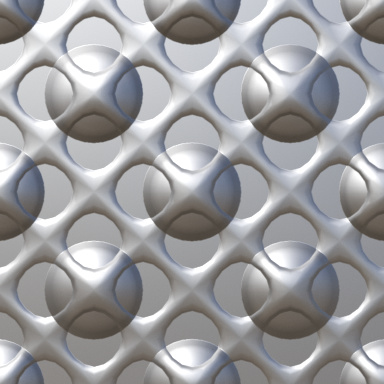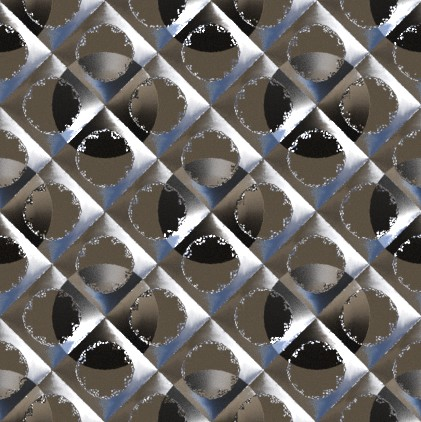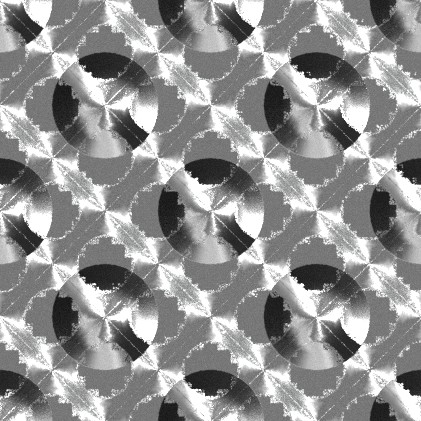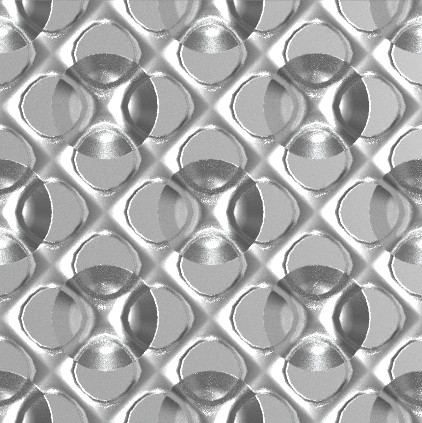# How Combine (Merge) Two Normal Output (Not Normal Map)?

No, this method produce wrong result.

When I calculate normals for some special situations, sometimes need merge two calculated normals. Convert Tangent Space after again convert World Space is too cost procedure, and I need a direct method.

When I say to use the MixRGB, is to do it directly with the world vectors!!! No transformation is needed.

I know it might seem strange to put vectors in the MixRGB, but the math inside is what you want:

The mix function take a factor and two vectors (a color is a vector in a rgb space, nothing more).

It calculates a result vector `VR = VA*(1-fac) + VB*fac`. And this is also a very simple way to interpolate (mix) between two vectors in world space.

There is one minor problem when VA == -VB, but this is very difficult to happen if your vectors belong to the same hemisphere (they are normal vectors, right?).

Now, if you want something other than interpolate between your both vectors, you need to clarify us. This thread has already a hand full of answers, and you keep saying they don’t fit… perhaps you need to explain exactly what you’re looking for (with examples, real problems, blend files, etc).

1 Like

Yes, I know, but this not produce right result, because this is increase Roughness of the surface and kill details.

Merged Normal MapMixed Normalsis this better??

EDIT: WRONG VERSION… see below.

This is result:sorry, I uploaded the wrong setup…

btw… which blender version are you using?

Not change… I’m use 2.80.

If you disconnect Mix factors and set “0.5”, result be better, but still wrong.

OK… Prior to 2.81, the Vector Math outputs are different (cross product returns a normalized vector, with it’s magnitude in the float output)…
here’s how it works in those versions:

1 Like

Result:Thanks to secrop. This node setup works properly.

This is Blend file:

Normal_Combine.7z (2.5 MB)

Edit: For properly result, disable “Normalize” node.

There is one problem: It produce little overexposed result.

Merged Normal Maps:Combined Normals:I didn’t use the same formula has in the setup for normal maps… the results aren’t supposed to be identical.Do you really want them to produce the same output?

I want same result, because “Merge Normal Map” formula produce perfect result (as possible as).

This formula works good for not roughness surfaces, but when increase roughness, this artifact occurs. I think this occurs because of “Cross Product”, maybe “floating point” problem. Example, fake anisotropy formula used in Far Cry 4 occurs same problem because of “Cross Product”.